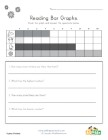These worksheets are the perfect introduction to bar graphs for kids. They are very simple and ask kids to use the bar graph in each worksheet to find the count of the different things featured in each.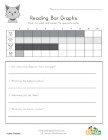Animal Themed Bar Graph Worksheet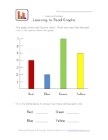Bar Graph Worksheet with Colors Theme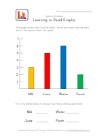Bar Graph Worksheet with Drinks Theme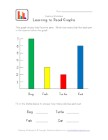Bar Graph Worksheet with Pets Theme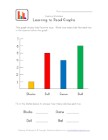Bar Graph Worksheet with Toys Theme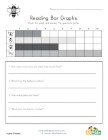Bug Themed Bar Graph Worksheet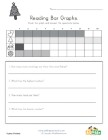Christmas Bar Graph Worksheet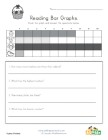Easter Bar Graph Worksheet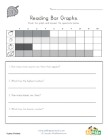Fall Bar Graph Worksheet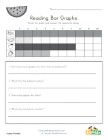Fruit Themed Bar Graph Worksheet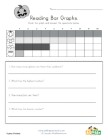Halloween Bar Graph Worksheet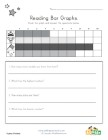Memorial Day Bar Graph Worksheet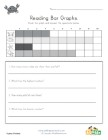Ocean Themed Bar Graph Worksheet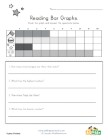Patriotic Bar Graph Worksheet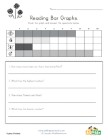Spring Bar Graph Worksheet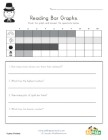St. Patrick's Day Bar Graph Worksheet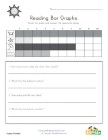Summer Bar Graph Worksheet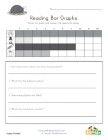Thanksgiving Bar Graph Worksheet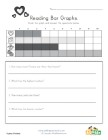Valentine's Day Bar Graph Worksheet